This is “Applying LIFO and Averaging to Determine Reported Inventory Balances”, section 9.5 from the book Business Accounting (v. 2.0). For details on it (including licensing), click here.

Has this book helped you? Consider passing it on:
Creative Commons supports free culture from music to education. Their licenses helped make this book available to you.
DonorsChoose.org helps people like you help teachers fund their classroom projects, from art supplies to books to calculators.

## 9.5 Applying LIFO and Averaging to Determine Reported Inventory Balances

### Learning Objectives

At the end of this section, students should be able to meet the following objectives:

1. Determine ending inventory and cost of goods sold using a periodic LIFO system.
2. Monitor inventory on an ongoing basis through a perpetual LIFO system.
3. Understand the reason that periodic LIFO and perpetual LIFO usually arrive at different figures.
4. Use a weighted average system to determine the cost of ending inventory and cost of goods sold.
5. Calculate reported inventory balances by applying a moving average inventory system.

## Applying LIFO

Question: LIFO reverses the FIFO cost flow assumption so that the last costs incurred are the first reclassified to cost of goods sold. How is LIFO applied to the inventory of an actual business? If the Mayberry Home Improvement Store adopted LIFO, how would the reported figures for its inventory have been affected by this decision?

Answer: Periodic LIFO. In a periodic system, only the computation of the ending inventory is directly affected by the choice of a cost flow assumption.Because ending inventory for one period becomes the beginning inventory for the next, application of a cost flow assumption does change that figure also. However, the impact is only indirect because the number is simply carried forward from the previous period. No current computation of beginning inventory is made based on the cost flow assumption in use. Thus, for this illustration, beginning inventory remains \$440 (4 units at \$110 each), and the number of units purchased is still eight with a cost of \$1,048. The figure that changes is the cost of the ending inventory. Four bathtubs remain in stock at the end of the year. According to LIFO, the last (most recent) costs are transferred to cost of goods sold. Only the cost of the first four units remains in ending inventory. That is \$110 per unit or \$440 in total.

Figure 9.8 Periodic LIFO—Bathtub Model WET-5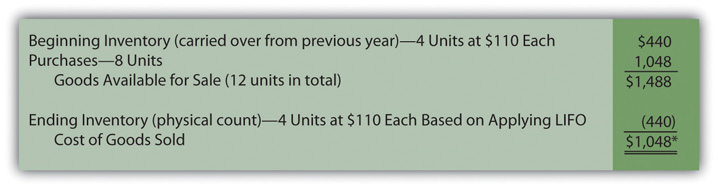*If the number of units bought during a period equals the number of units sold (as is seen in this example), the quantity of inventory remains unchanged. In a periodic LIFO system, beginning inventory (\$440) is then the same as ending inventory (\$440) so that cost of goods sold (\$1,048) equals the amount spent during the period to purchase inventory (\$1,048). For that reason, company officials can easily keep track of gross profit during the year by subtracting purchases from revenues.

If Mayberry Home Improvement Store uses a periodic LIFO system, gross profit for the year will be reported as \$902 (revenue of \$1,950 less cost of goods sold of \$1,048).

Note here that the anticipated characteristics of LIFO are present. Ending inventory of \$440 is lower than that reported by FIFO (\$558). Cost of goods sold (\$1,048) is higher than under FIFO (\$930) so that reported gross profit (and, hence, net income) is lower by \$118 (\$1,020 for FIFO versus \$902 for LIFO).

### Test Yourself

Question:

The Lowenstein Widget Company starts the year with 24,000 widgets costing \$3 each. During the year, the company bought another 320,000 widgets for a total of \$1,243,000. Within these figures was the acquisition of 20,000 widgets for \$4.10 each on December 26 and 15,000 widgets for \$4.00 each on December 18. Those were the last two purchases of the year. On December 31, the company took a physical count and found 21,000 widgets still on hand. If a periodic LIFO cost flow assumption is applied, what amount is reported on the income statement for cost of goods sold?

1. \$1,249,000
2. \$1,252,000
3. \$1,256,000
4. \$1,264,000

The correct answer is choice b: \$1,252,000.

Explanation:

Beginning inventory is \$72,000 (24,000 units at \$3 each) and purchases total \$1,243,000. Cost of goods available is the total or \$1,315,000. With LIFO, the 21,000 units on hand had the \$3 cost of the first items. Total cost for ending inventory is \$63,000. Subtracting this balance from goods of available for sale (\$1,315,000 less \$63,000) gives cost of goods sold of \$1,252,000. A LIFO liquidation took place since the inventory declined. That has no impact on the answer but is disclosed.

Perpetual LIFO. The mechanical structure for a perpetual LIFO system is the same as that demonstrated previously for perpetual FIFO except that the most recent costs are moved into cost of goods sold at the time of each sale (points A, B, and C).

Figure 9.9 Perpetual LIFO—Bathtub Model WET-5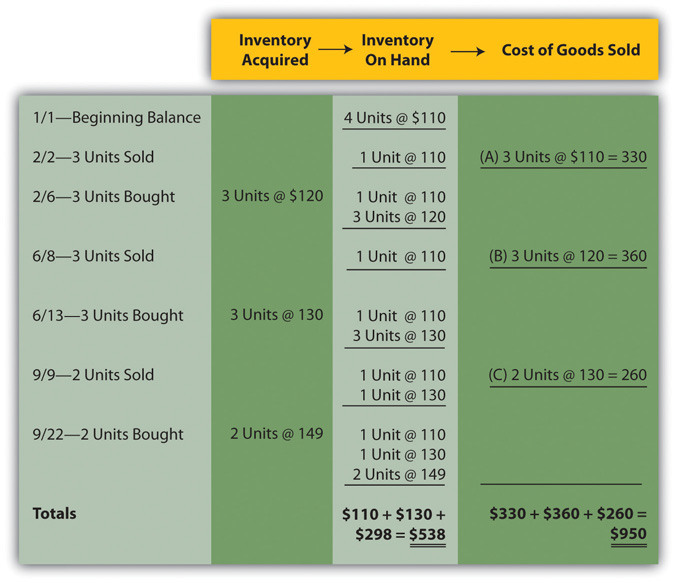Once again, the last cell at the bottom of the “inventory on hand” column contains the asset figure to be reported on the balance sheet (a total of \$538) while the summation of the “cost of goods sold” column provides the amount to be shown on the income statement (\$950).

As can be seen here, periodic and perpetual LIFO do not necessarily produce identical numbers.

periodic LIFO: ending inventory \$440 and cost of goods sold \$1,048 perpetual LIFO: ending inventory \$538 and cost of goods sold \$950

Although periodic and perpetual FIFO always arrive at the same results, balances reported by periodic and perpetual LIFO frequently differ. The first cost incurred in a period (the cost transferred to expense under FIFO) is the same regardless of the date of sale. However, the identity of the last or most recent cost (expensed according to LIFO) depends on the perspective.

To illustrate, note that two bathtubs were sold on September 9 by the Mayberry Home Improvement Store. Perpetual LIFO immediately determines the cost of this sale and reclassifies the amount to expense. On that date, the cost of the most recent two units (\$130 each) came from the June 13 purchase. In contrast, a periodic LIFO system makes this same determination but not until December 31. As viewed from year’s end, the last bathtubs had a cost of \$149 each. Although these items were bought on September 22, after the final sale, their costs are included in cost of goods sold when applying periodic LIFO.

Two bathtubs were sold on September 9, but the identity of the specific costs to be transferred (when using LIFO) depends on the date on which the determination is made. A periodic system views the costs from the perspective of the end of the year. A perpetual system determines the expense immediately when each sale is made.

### Test Yourself

Question:

A company starts the year with 100 units of inventory costing \$9 each. Those units are all sold on June 23. Another 100 are bought on July 6 for \$11 each. On November 18, 70 of these units are sold. On December 16, fifty units are bought for \$15 each bringing the total to eighty (100 – 100 + 100 – 70 + 50). If a perpetual LIFO system is used, what is the cost of these eighty units in ending inventory?

1. \$720
2. \$960
3. \$1,080
4. \$1,200

The correct answer is choice c: \$1,080.

Explanation:

In a perpetual LIFO system, the entire opening cost is transferred to cost of goods sold on June 23. On November 18, the cost of seventy units bought on July 6 is also transferred. That leaves thirty units at \$11 each (\$330) plus the December 16 purchase of fifty units at \$15 each or \$750. Ending inventory then has a total of \$1,080 (\$330 plus \$750). The reclassification takes place each time at the point of the sale.

In practice, many companies are unlikely to use perpetual LIFO inventory systems. They are costly to maintain and, as has been discussed previously, provide figures of dubious usefulness. For that reason, companies often choose to maintain a perpetual FIFO system for internal decision making and then use the periodic LIFO formula at the end of the year to convert the numbers for external reporting purposes.

For example, The Kroger Co. presented the following balances on its January 29, 2011, balance sheet:

• FIFO inventory: \$5,793 million
• LIFO reserve: (827) million

Kroger apparently monitors its inventory on a daily basis using FIFO and arrived at a final cost of \$5,793 million. However, at the end of that year, the company took a physical inventory and applied the LIFO cost flow assumption to arrive at a reported balance that was \$827 million lower. The reduced figure was used for reporting purposes because of the LIFO conformity rule. However, investors and creditors could still see that ending inventory actually had a current cost of \$5,793 million.

## Applying Averaging as a Cost Flow Assumption

Question: Not surprisingly, averaging follows a path similar to that of the previous examples. Costs are either moved to cost of goods sold at the end of the year (periodic or weighted average) or at the time of each new sale (perpetual or moving average). The only added variable to this process is the calculation of average cost. In the operation of an averaging system, when and how is the average cost of inventory determined?

Answer: Periodic (weighted) average. In the problem being examined here, Mayberry Home Improvement Store eventually held twelve bathtubs. Four of these units were on hand at the start of the year and the other eight were acquired during the period. The beginning inventory cost \$440 and the new purchases were bought for a total of \$1,048.

These twelve units had a total cost of \$1,488 (\$440 + \$1,048) or \$124 per bathtub (\$1,488/12 units). When applying a weighted average system, this single average for the entire period is the basis for both the ending inventory and cost of goods sold to be reported in the financial statements. No item actually cost \$124 but that average is applied to all units.

Figure 9.10 Periodic (Weighted) Average—Bathtub Model WET-5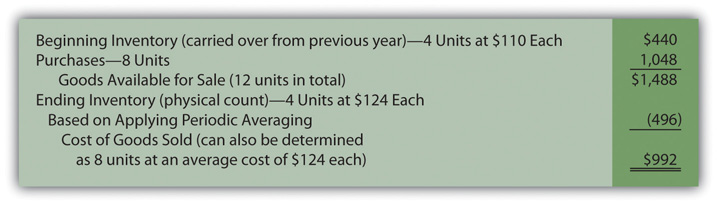Perpetual (moving) average. In this final approach to maintaining and reporting inventory, each time that a company buys inventory at a new price, the average cost is recalculated. Therefore, a moving average system must be programmed to update the average whenever additional merchandise is acquired.

In Figure 9.11 "Perpetual (Moving) Average—Bathtub Model WET-5", a new average is computed at points D, E, and F. This figure is found by dividing the number of units on hand after the new purchase into the total cost of those items. For example, at point D, the company now has four bathtubs. One cost \$110 while the other three were newly acquired for \$120 each or \$360 in total. Total cost was \$470 (\$110 + \$360) for these four units for an updated average of \$117.50 (\$470/4 units). That average is used until the next purchase is made on June 13. The applicable average at the time of sale is transferred from inventory to cost of goods sold at points A (\$110.00), B (\$117.50), and C (\$126.88).

Figure 9.11 Perpetual (Moving) Average—Bathtub Model WET-5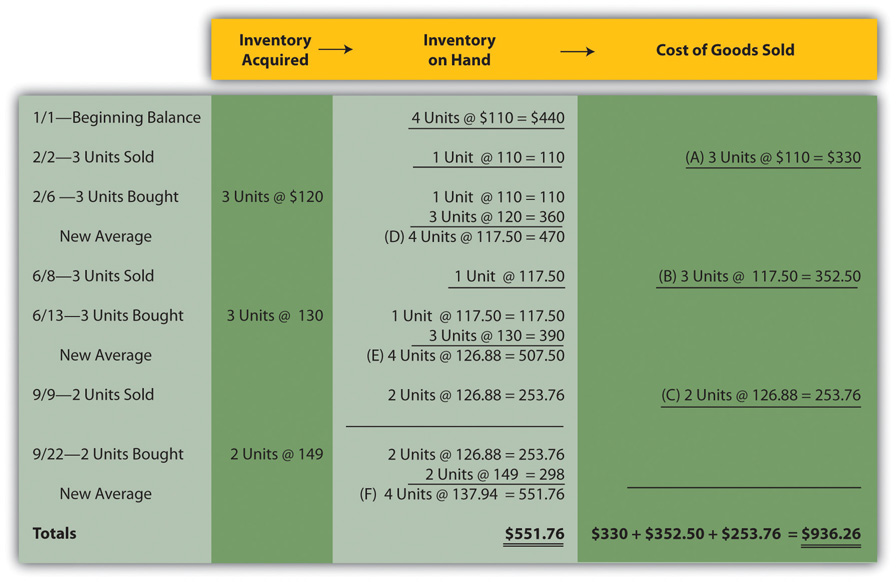Summary. The six inventory systems shown here for Mayberry Home Improvement Store provide a number of distinct pictures of ending inventory and cost of goods sold. As stated earlier, these numbers are all fairly presented but only in conformity with the specified principles being applied. Interestingly, gross profit ranges from \$902.00 to \$1,020.00 based on the system applied by management.

Figure 9.12 Reported Balances for Six Inventory Systems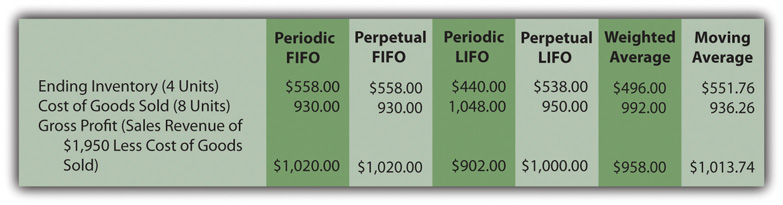### Test Yourself

Question:

A company begins the new year with twenty-five units of inventory costing \$12 each. In February, fifteen of these units are sold. At the beginning of May, fifty new units are acquired at \$15 each. Finally, in August, forty more units are sold. On December 31, a physical inventory count is taken and twenty units are still on hand. Thus, no units were lost or stolen (25 units – 15 sold + 50 bought – 40 sold = 20 units remaining). If a weighted average system is used, what is the cost to be reported for those twenty units of inventory?

1. \$250
2. \$260
3. \$270
4. \$280

The correct answer is choice d: \$280.

Explanation:

In a weighted (or periodic) averaging system, the average for the year is not determined until financial statements are to be produced. Beginning inventory was \$300 (twenty-five units for \$12 each) and purchases were \$750 (fifty units for \$15 each) for a total of seventy-five units costing \$1,050 (\$300 + \$750). That gives an average of \$14 per unit (\$1,050 cost/75 units). With this assumption, the cost assigned to the ending inventory of 20 units is \$280 (20 units at \$14 each).

### Test Yourself

Question:

A company begins the new year with twenty-five units of inventory costing \$12 each. In February, fifteen of these units are sold. At the beginning of May, fifty new units are acquired at \$15 each. Finally, in August, forty more units are sold. On December 31, a physical inventory count is taken and twenty units are still on hand. Thus, no units were lost or stolen (25 units – 15 sold + 50 bought – 40 sold = 20 units remaining). If a moving average system is used, what is the cost to be reported for those twenty units?

1. \$260
2. \$270
3. \$280
4. \$290